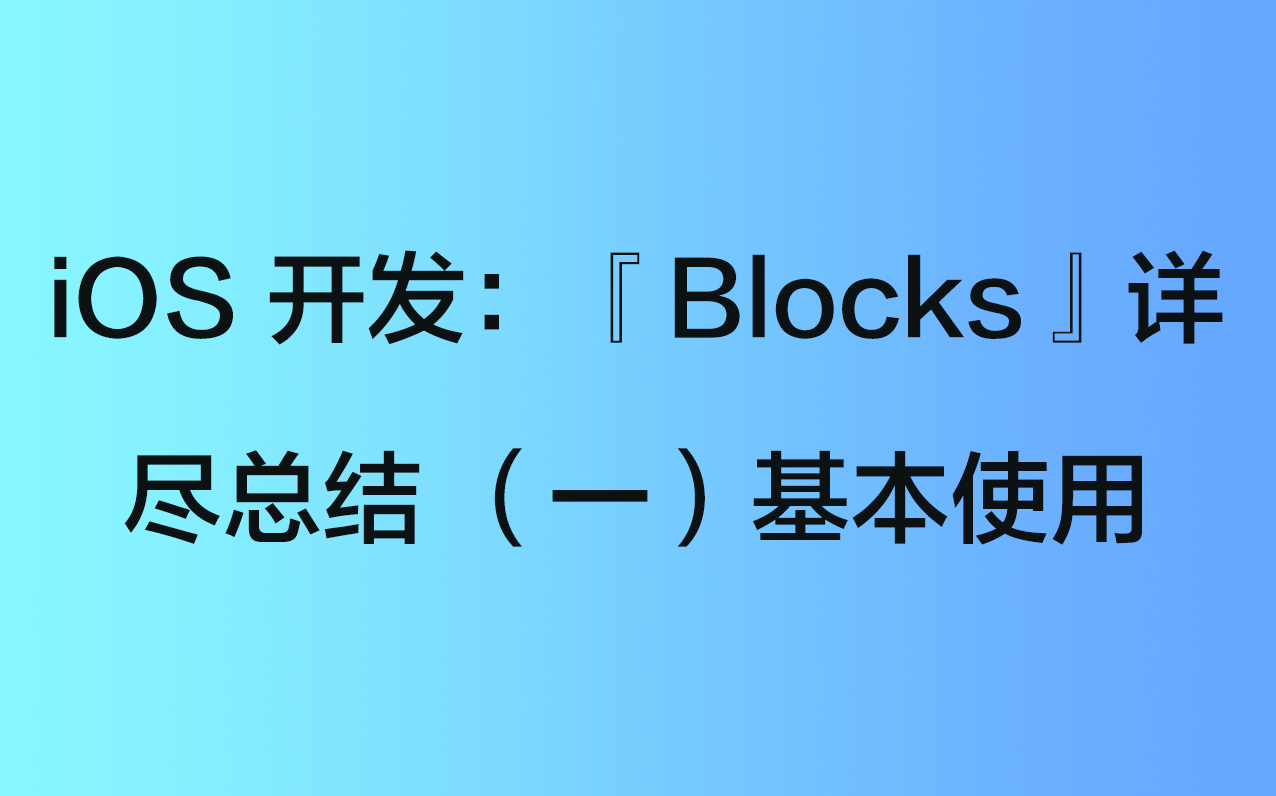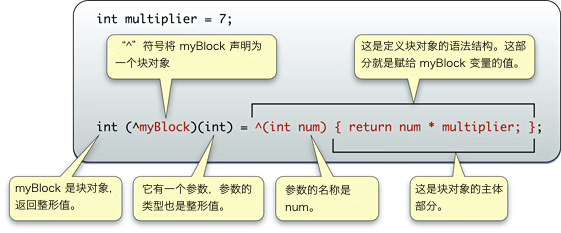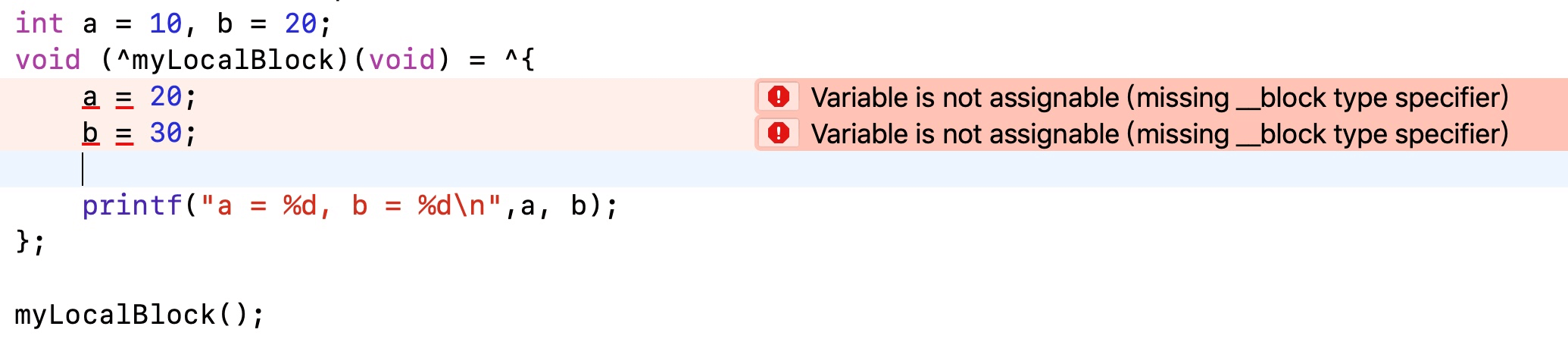# iOS 开发：「Blocks」详尽总结 （一）基本使用

5.959k 字  |  20 分钟

1. 什么是 Blocks
2. Blocks 变量语法
3. Blocks 变量的声明与赋值
4. Blocks 变量截获局部变量值特性
5. 使用 __block 说明符
6. Blocks 变量的循环引用以及如何避免# 1. 什么是 Blocks ？

Blocks 也被称作 闭包代码块。展开来讲，Blocks 就是一个代码块，把你想要执行的代码封装在这个代码块里，等到需要的时候再去调用。

## 1.1 局部变量

int x, y; // x，y 为全局变量

int fun(int a) {
int b, c; //a，b，c 为局部变量
return a+b+c;
}

int main() {
int m, n; // m，n 为局部变量
return 0;
}

1. 我们在开始位置定义了变量 x 和 变量 y。 x 和 y 都是全局变量。它们的作用域默认是整个程序，也就是所有的源文件，包括 .c 和 .h 文件。
2. 而我们在 fun() 函数中定义了变量 a、变量 b、变量 c。它们的作用域是 fun() 函数。只能在 fun() 函数内部使用，离开 fun() 函数就是无效的。
3. 同理，main() 函数中的变量 m、变量 n 也只能在 main() 函数内部使用。

## 1.2 匿名函数

int fun(int a);

fun 就是这个函数的名称，在调用的时候必须要使用该函数的名称 fun 来调用。

int result = fun(10);

int (*funPtr)(int) = &fun;
int result = (*funPtr)(10);

# 2. Blocks 变量语法

int multiplier = 7;
int (^ myBlock)(int)= ^(int num) {
return num * multiplier;
};^ 返回值类型 (参数列表) { 表达式 };

^ int (int count) { return count + 1; };

Blocks 规定可以省略好多项目。例如：返回值类型参数列表。如果用不到，都可以省略。

## 2.1 省略返回值类型： ^ (参数列表) { 表达式 };

^ (int count) { return count + 1; };

^ void (int count)  { printf("%d\n", count); };    // 返回值类型使用 void
^ (int count) { printf("%d\n", count); };    // 省略返回值类型

## 2.2 省略参数列表 ^ 返回值类型 (void) { 表达式 };

^ int (void) { return 1; };    // 参数列表使用 void
^ int { return 1; };    // 省略参数列表类型

## 2.3 省略返回值类型、参数列表： ^ { 表达式 };

^ { printf("Blocks"); }; 

# 3. Blocks 变量的声明与赋值

## 3.1 Blocks 变量的声明与赋值语法

Blocks 变量的声明与赋值语法可以总结为：

int (^blk) (int)  = ^(int count) { return count + 1; };
int (^blk1) (int);    // 声明变量名为 blk1 的 Blocks 变量
blk1 = blk;        // 将 blk 赋值给 blk1

Blocks 变量的声明语法有点复杂，其实我们可以和 C 语言函数指针的声明类比着来记。

Blocks 变量的声明就是把声明函数指针类型的变量 * 变为 ^

//  C 语言函数指针声明与赋值
int func (int count) {
return count + 1;
}
int (*funcptr)(int) = &func;

// Blocks 变量声明与赋值
int (^blk) (int)  = ^(int count) { return count + 1; };

## 3.2 Blocks 变量的声明与赋值的使用

### 3.2.1 作为局部变量：返回值类型 (^变量名) (参数列表) =  返回值类型 (参数列表) { 表达式 };

// Blocks 变量作为本地变量
- (void)useBlockAsLocalVariable {
void (^myLocalBlock)(void) = ^{
NSLog(@"useBlockAsLocalVariable");
};

myLocalBlock();
}


### 3.2.2 作为带有 property 声明的成员变量：@property (nonatomic, copy) 返回值类型 (^变量名) (参数列表);

/* Blocks 变量作为带有 property 声明的成员变量 */
@property (nonatomic, copy) void (^myPropertyBlock) (void);

// Blocks 变量作为带有 property 声明的成员变量
- (void)useBlockAsProperty {
self.myPropertyBlock = ^{
NSLog(@"useBlockAsProperty");
};

self.myPropertyBlock();
}

### 3.2.3 作为 OC 方法参数：- (void)someMethodThatTaksesABlock:(返回值类型 (^)(参数列表)) 变量名;

// Blocks 变量作为 OC 方法参数
- (void)someMethodThatTakesABlock:(void (^)(NSString *)) block {
block(@"someMethodThatTakesABlock:");
}

### 3.2.4 调用含有 Block 参数的 OC方法：[someObject someMethodThatTakesABlock:^返回值类型 (参数列表) { 表达式}];

// 调用含有 Block 参数的 OC方法
- (void)useBlockAsMethodParameter {
[self someMethodThatTakesABlock:^(NSString *str) {
NSLog(@"%@",str);
}];
}

### 3.2.5 作为 typedef 声明类型：

typedef 返回值类型 (^声明名称)(参数列表);

// Blocks 变量作为 typedef 声明类型
- (void)useBlockAsATypedef {
typedef void (^TypeName)(void);

// 之后就可以使用 TypeName 来定义无返回类型、无参数列表的 block 了。
TypeName myTypedefBlock = ^{
NSLog(@"useBlockAsATypedef");
};

myTypedefBlock();
}

# 4. Blocks 变量截获局部变量值特性

// 使用 Blocks 截获局部变量值
- (void)useBlockInterceptLocalVariables {
int a = 10, b = 20;

void (^myLocalBlock)(void) = ^{
printf("a = %d, b = %d\n",a, b);
};

myLocalBlock();    // 打印结果：a = 10, b = 20

a = 20;
b = 30;

myLocalBlock();    // 打印结果：a = 10, b = 20
}

这就是 Blocks 变量截获局部变量值的特性。

# 5. 使用 __block 说明符// 使用 __block 说明符修饰，更改局部变量值
- (void)useBlockQualifierChangeLocalVariables {
__block int a = 10, b = 20;
void (^myLocalBlock)(void) = ^{
a = 20;
b = 30;

printf("a = %d, b = %d\n",a, b);  // 打印结果：a = 20, b = 30
};

myLocalBlock();
}

# 6. Blocks 变量的循环引用以及如何避免

/* —————— retainCycleBlcok.m —————— */
#import <Foundation/Foundation.h>
#import "Person.h"
int main() {
Person *person = [[Person alloc] init];
person.blk = ^{
NSLog(@"%@",person);
};

return 0;
}

/* —————— Person.h —————— */
#import <Foundation/Foundation.h>

typedef void(^myBlock)(void);

@interface Person : NSObject
@property (nonatomic, copy) myBlock blk;
@end

/* —————— Person.m —————— */
#import "Person.h"

@implementation Person

@end

## 6.1 ARC 下，通过 __weak 修饰符来消除循环引用

int main() {
Person *person = [[Person alloc] init];
__weak typeof(person) weakPerson = person;

person.blk = ^{
NSLog(@"%@",weakPerson);
};

return 0;
}

## 6.2 MRC 下，通过 __block 修饰符来消除循环引用

MRC 下，是不支持 weak 修饰符的。但是我们可以通过 block 来消除循环引用。

int main() {
Person *person = [[Person alloc] init];
__block typeof(person) blockPerson = person;

person.blk = ^{
NSLog(@"%@", blockPerson);
};

return 0;
}

# 评论（没有评论）# 程序员充电站微信支付支付宝